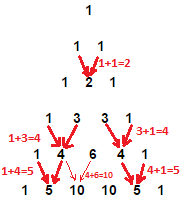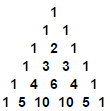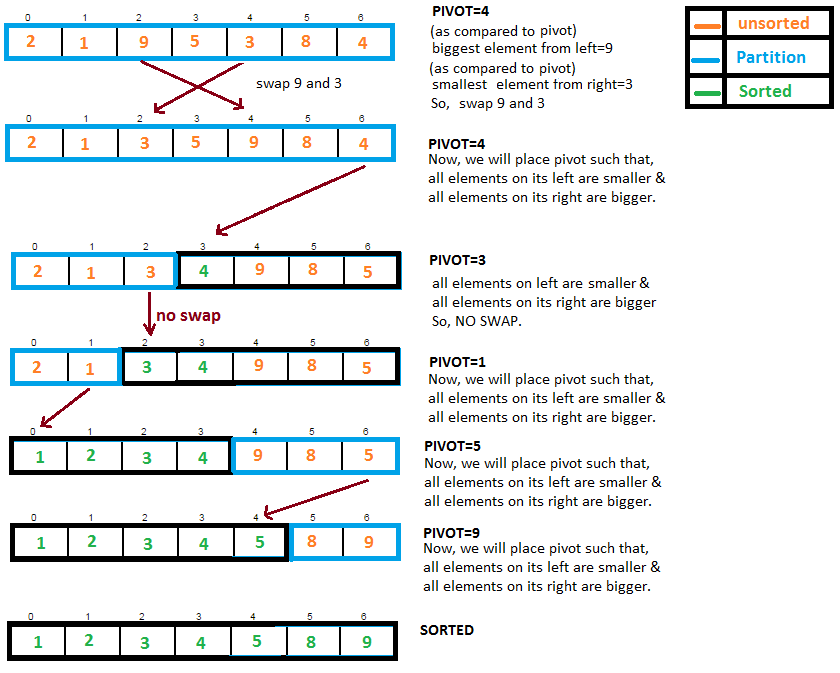### Pattern 8 - Pascal’s triangle - Pyramid generation program in javaWrite a program to generate this Pascal’s triangle in java.>Each number present in row is sum of the left & right number in the above row.
>If there is no number in number in above, number is assumed as 0.>First and last number in row is always 1.
>Sum of numbers in each row is equal to sum of numbers in above row.

Must read:Quick Sort.

Example / Full Program/SourceCode to generate Pascal’s triangle in java. >
 package pyramid; /* */ import java.util.Scanner; /** Copyright (c), AnkitMittal  JavaMadeSoEasy.com */ public class Pyramid8Pascal {    public static void main(String[] args) {                     Scanner scanner = new Scanner(System.in);           System.out.print("Enter no of rows in Pascal's triangle : ");           int rows = scanner.nextInt();           int nextNumber;           System.out.println("");           for (int i = 0; i < rows; i++) {                  nextNumber = 1;                                   for(int k=0; k<(rows-i)*2; k++)   //create (rows-i)*2  spaces, for initial spacing.                        System.out.print(" ");                                   for (int j = 0; j <= i; j++) {                        System.out.format("%4d", nextNumber); // %4d creates 4 space between number.                        nextNumber = nextNumber * (i - j) / (j + 1);                  }                  System.out.println();           }    } } /* OUTPUT Enter no of rows in Pascal's triangle : 6                  1                1   1            1   2   1           1   3   3   1         1   4   6   4   1      1   5  10  10   5   1 */

So wrote a program to generate this Pascal’s triangle in java.

Having any doubt? or you you liked the tutorial! Please comment in below section.